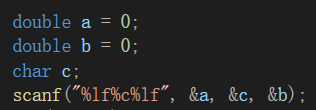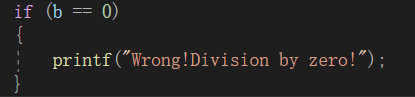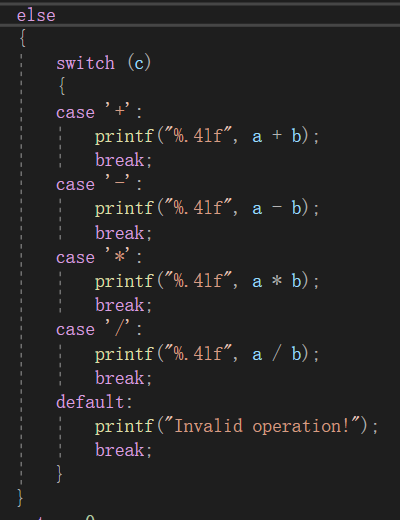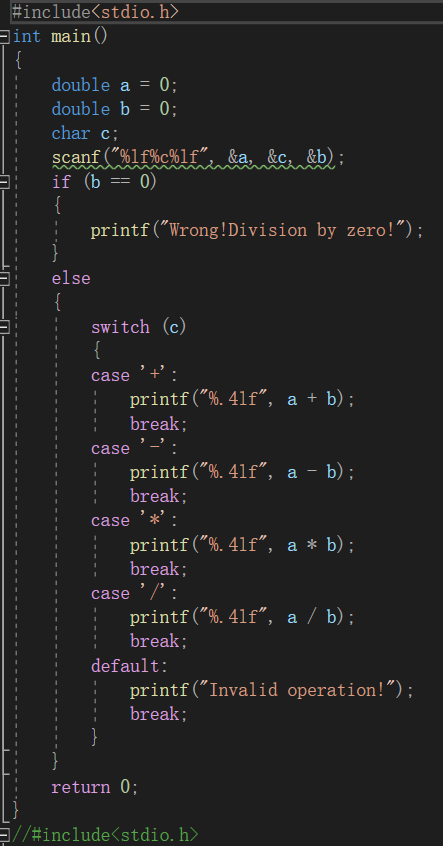• c语言计算器代码加减乘除
千次阅读
2021-11-24 21:15:33

#include<stdio.h>
#include<stdlib.h>
{
printf("           1.加法     2.减法      \n ");
printf("           3.乘法     4.除法      \n");
printf("                 0.退出           \n");
}
int  jia(int x, int y)                //加法实现
{
return x + y;
}
int  jian(int x, int y)              //减法实现
{
return x - y;
}
int  cheng(int x, int y)          //乘法实现
{
return x * y;
}
int  chu(int x, int y)                //除法实现
{
return x / y;
}
int main()
{
int a, b, num, ret;
int (*p)() = { 0,jia,jian,cheng,chu };
while (1)
{
printf("请选择：");
scanf_s("%d", &num);
if (num <= 4 && num >= 1)
{
printf("请输入两个数：");
scanf_s("%d %d", &a, &b);
ret = (*p[num])(a, b);
printf("计算得出：%d\n", ret);
}
else if (num == 0)
{
printf("退出程序\n");
}
else
{
printf("输入有误\n");
}
}
system("pause");
return 0;
return 0;
}

更多相关内容
• printf("计算器\n"); printf(“请输入一种算法\n”); printf("\n"); printf("by：兴\n"); printf("**********\n”); } int add(data1,data2){ int z; z = data1+data2; return z; } int Jian(data1,data2){ int z; z...

#include<stdio.h>
void tips(){
printf(“\n");
printf("计算器
\n");
printf(“请输入一种算法\n”);
printf("
\n");
printf("by：兴\n");
printf("
**********\n”);
}
int z;
z = data1+data2;
return z;
}
int Jian(data1,data2){
int z;
z = data1-data2;
return z;
}
int Cheng(data1,data2){
int z;
z = data1*data2;
return z;
}
float Chu(data1,data2){
float z;
z = (float)data1/data2;
return z;
}

int main()
{
int data1;
int data2;
int result;
float result2;
char suanFa;
int mark = 0;
while(1)
{
tips();
if(mark != 0)
{
getchar(); **//用处吸收回车，回 车也是一种字符，这里的作用是把输入data2之后的回车吸收掉
}
mark = 1;
scanf("%c",&suanFa);

switch(suanFa)
{
case'+':
printf("你选择了加法");
printf("请输入第一个数\n");
scanf("%d",&data1);
printf("请输入第二个数\n");
scanf("%d",&data2);
printf("加法结果为：%d\n",result);
break;
case'-':
printf("你选择了减法");
printf("请输入第一个数\n");
scanf("%d",&data1);
printf("请输入第二个数\n");
scanf("%d",&data2);
result = Jian(data1,data2);
printf("减法结果为：%d\n",result);
break;
case'*':
printf("你选择了乘法");
printf("请输入第一个数\n");
scanf("%d",&data1);
printf("请输入第二个数\n");
scanf("%d",&data2);
result = Cheng(data1,data2);
printf("乘法结果为：%d\n",result);
break;
case'/':
printf("你选择了除法");
printf("请输入第一个数\n");
scanf("%d",&data1);
printf("请输入第二个数\n");
scanf("%d",&data2);
result2 = Chu(data1,data2);
printf("除法结果为：%f\n",result);
break;
default:
printf("你选择了鬼算法");
break;
}
}
system("pause");
return 0;


}

展开全文• 然后剩下就根据字符C，也就是看加减乘数，我们采用switch语句与case结合（一直用if也是可以） 所以总代码为： #include<stdio.h> int main() { double a = 0; double b = 0; char c; scanf("%lf%c%...

首先我们定义三个变量，因为有小数所以double类型因为除数不能为0，所以先设置一个if语句然后剩下就根据字符C，也就是看加减乘数，我们采用switch语句与case结合（一直用if也是可以）所以总代码为：#include<stdio.h>
int main()
{
double a = 0;
double b = 0;
char c;
scanf("%lf%c%lf", &a, &c, &b);
if (b == 0)
{
printf("Wrong!Division by zero!");
}
else
{
switch (c)
{
case '+':
printf("%.4lf", a + b);
break;
case '-':
printf("%.4lf", a - b);
break;
case '*':
printf("%.4lf", a * b);
break;
case '/':
printf("%.4lf", a / b);
break;
default:
printf("Invalid operation!");
break;
}
}
return 0;
}

展开全文• C语言计算器程序，可以实现加减乘除等功能，有界面，有注释，可以运行。可以计算小数，计算没有错误。没有括号运算。
• 计算器的实现思路菜单加减乘除内容的实现 思路 菜单 看了看计算器想了下计算机只能实现加法所以用了补码和反码来实现减乘除，编译器VS2013那么我们虽然造不出来显示中的计算器那么我们可以在代码中亲送的实现首先...

# 思路

### 菜单

看了看计算器想了下计算机只能实现加法所以用了补码和反码来实现减乘除，编译器VS2013那么我们虽然造不出来显示中的计算器那么我们可以在代码中亲送的实现首先我们需要有菜单这样

void menu()
{
printf("--------------------\n");
printf("    请输入你的选择 \n");
printf("        1.+       \n");
printf("        2.-       \n");
printf("        3.*       \n");
printf("        4./       \n");
printf("        5./0      \n");
printf("--------------------\n");
}


### 加减乘除内容的实现

do
{
scanf("%d", &select);   //输入你的选项
printf("请输入计算值:");
scanf("%f%f", &i, &j);    //输入要计算的数值
switch (select)
{
case 1://实现加法功能
printf("%f+%f=%f\n", i, j, num = i + j);
break;
case 2: //实现减法功能
printf("%f-%f=%f\n", i, j, num = i - j);
break;
case 3:// 实现乘法功能
printf("%f*%f=%f\n", i, j, num = i*j);
break;
case 4:  //实现除法功能
printf("%f/%f=%f\n", i, j, num = i / j);
break;
case 5：
exit（0）；
default:
printf("错误输入，请重新输入");
break;
}

} while (select);

展开全文• 输入为数字和算术符号组成的字符串，程序会根据运算级处理后输出结果，这是前段时间写的了，没有添加输入字母报错功能，当时我认为计算器上一般也没有字母按键，所以就没有写，但是现在想想计算器和这个代码实现的...
• 计算器加减乘除的实现 int Add(int x, int y) { return x + y; } int Sub(int x, int y) { return x - y; } int Mul(int x, int y) { return x * y; } int Div(int x, int y) { return x / y; } int main() { ...
• C语言和C++在VS2010实现，计算器加减乘除，括号优先级
• 速算,简易计算器c语言代码,可实现加减乘除#include"stdio.h"#include"math.h"#include"stdlib.h"struct optrstyle{int top;char stack;}struct opndstyle{int top;int stack;}void main(){char ch;while(1){...
• 基本上每个人都会使用计算器，那么如何利用C语言设计出一个自己的计算器呢？其实并不难，我们只需要掌握一些C语言基本的知识点就可以完成，下面我们来讲解如何利用循环语句和函数调用等简单知识点来实现设计出一个...
• 编写程序简单实现计算器加减乘除功能（函数指针数组的写法）
• c语言计算器代码（有注释），可实现二、十、十六等多进制的加减乘除运算，可实现界面刷新、返回、退出等多项功能c语言课程设计
• 写一个计算器C语言版本），可以求出：整数的，乘，除四则运算
• c语言实现的整数加减乘除运算，实现竖式表达，可以连续运算，采用栈的方式完成四则运算中的先乘除后加减。
• 简单计算器 问题描述 实现两个整数的相加,相减,相乘和相除操作,使用转移表. 主要函数 相加 int add(int a, int b){ return a + b; } 相减 int sub(int a, int b){ return a - b; } 相乘 int mul(int a, ...
• printf("*############高级计算器############* \n"); printf("************************************ \n"); printf("* ①加法运算 * \n"); printf("* ②减法运算 * \n"); printf("* ③乘法运算 * \n"); printf("* ...
• 该楼层疑似违规已被系统折叠隐藏此楼查看此楼case 0x7b: dengyu=1; //key=11; //wei++; break; } while(temp!=0xf0) { temp=P3; temp=temp&0xf0; ...
• 方法三：使用main函数的参数，实现一个整数计算器，程序可以接受三个参数，第一个参数“-a”选项执行加法，“-s”选项执行减法，“-m”选项执行乘法，“-d”选项执行除法，后面两个参数为操作数。 例如：命令行参数...
• C语言 计算器程序 支持 加减乘除（）^运算（收集） （没有图形界面）C语言 计算器
• `c #include<stdio.h> #include<stdlib.h> //加法 void Add(float a,float b) { printf("%f\n\n", a + b); } //减法 void Sub(float a, float b) { printf("%f\n\n", a - b);...void Mul(flo...C语言入门
• 学 海 无 涯 #include<stdio.h> double jjcc(double st[],int k){ for(int j=1;j;j++){ if(st[j]=='||st[j]=='/){ if(st[j]==) st[j-1]=st[j-1]*st[j+1]; else st[j-1]=st[j-1]/st[j+1]; int y=j;...
• import java.util.ArrayList;import java.util.List;import java.util.Stack;public class Calculator {/*** @param args*/public static void main(String[] args) throws Exception {Listhouzhuishi = houzhui("(0...
• ;学 海 无 涯 sum1=(st1[i]-48)+sum1*10; i++; if(st1[i]==){ i++; while(st1[i]>47&st1[i]){ k=k*0.1; sum2=(st1[i]-48*k+sum2; i++; } } st2[c]=sum1+sum2; } else{ st2[++c]=st1[i]; i++;... } } printf
• #includedouble jjcc(double st[],int k){ for(int j=1;jif(st[j]=='*'||st[j]=='/'){if(st[j]=='*')st[j-1]=st[j-1]*st[j+1];elsest[j-1]=st[j-1]/st[j+1];int y=j;int n=0;while(nst[y]=st[y+2];...
• 编写程序的目的就是使程序有他应用的地方，编写一个简单的计算器来实现我们计算的目的。  利用swich case 语句和循环结构来实现简单程序的编写。利用选择语句来进行输入的选择，然后利用所输入的数字的不同进行...
• 本程序对于初学C语言的同学很有帮助，#include "iostream" using namespace std; int main( void ) { double numbera, numberb, result; char operate; try { //输入操作数和运算符 cout请输入第一个操作...C语言 计算器
• c语言实现整数加减乘除计算器，采用非栈的方式处理先乘除后加减的功能 。实现竖式输出，支持连续运算功能，...